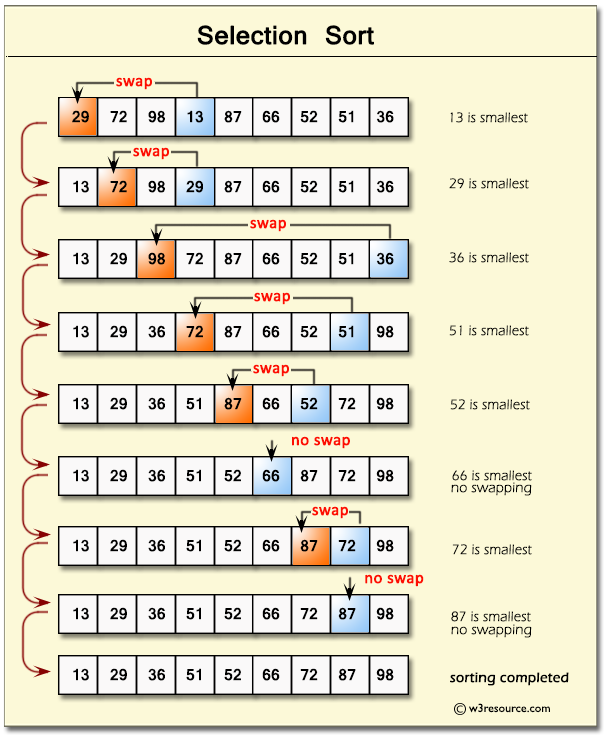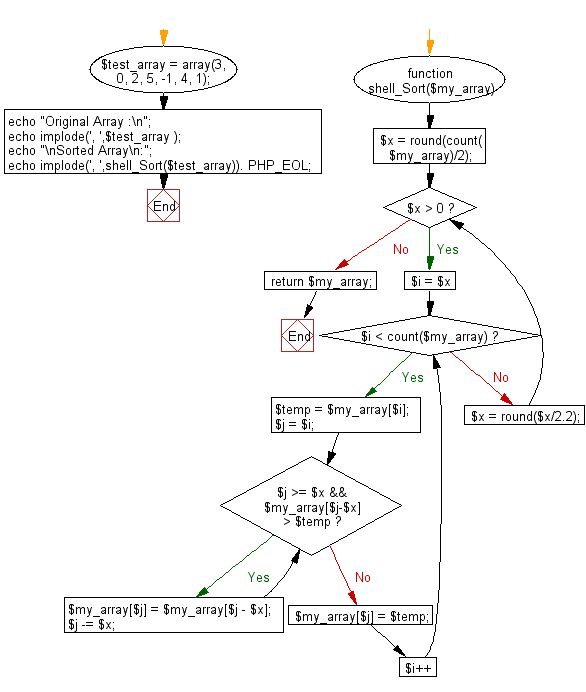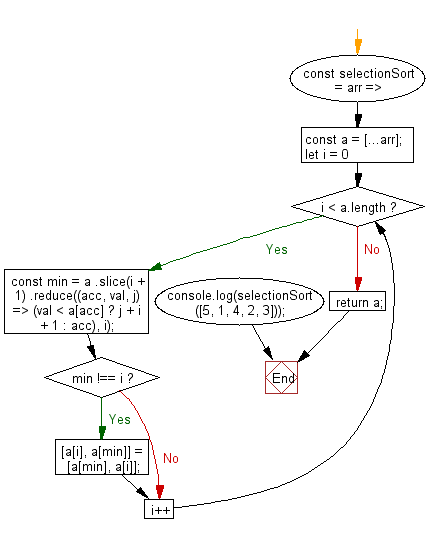# JavaScript Sorting Algorithm: Sorts an array of numbers, using the selection sort algorithm

## JavaScript Sorting Algorithm: Exercise-5 with Solution

Write a JavaScript program to sort a list of elements using the Selection sort algorithm.

The selection sort improves on the bubble sort by making only one exchange for every pass through the list.

Pictorial Presentation: Selection SortSample Solution-1:

JavaScript Code:

``````// Selection sort with O(n^2) time complexity

function Selection_Sort(arr, compare_Function) {

function compare(a, b) {
return a - b;
}
var min = 0;
var index = 0;
var temp = 0;

//{Function} compare_Function Compare function
compare_Function = compare_Function || compare;

for (var i = 0; i < arr.length; i += 1) {
index = i;
min = arr[i];

for (var j = i + 1; j < arr.length; j += 1) {
if (compare_Function(min, arr[j]) > 0) {
min = arr[j];
index = j;
}
}

temp = arr[i];
arr[i] = min;
arr[index] = temp;
}

//return sorted arr
return arr;
}

console.log(Selection_Sort([3, 0, 2, 5, -1, 4, 1], function(a, b) { return a - b; }));
console.log(Selection_Sort([3, 0, 2, 5, -1, 4, 1], function(a, b) { return b - a; }));
```
```

Sample Output:

```[-1,0,1,2,3,4,5]
[5,4,3,2,1,0,-1]
```

Flowchart:Sample Solution-2:

• Use the spread operator (...) to clone the original array, arr.
• Use a for loop to iterate over elements in the array.
• Use Array.prototype.slice() and Array.prototype.reduce() to find the index of the minimum element in the subarray to the right of the current index and perform a swap, if necessary.

JavaScript Code:

``````const selectionSort = arr => {
const a = [...arr];
for (let i = 0; i < a.length; i++) {
const min = a
.slice(i + 1)
.reduce((acc, val, j) => (val < a[acc] ? j + i + 1 : acc), i);
if (min !== i) [a[i], a[min]] = [a[min], a[i]];
}
return a;
};

console.log(selectionSort([5, 1, 4, 2, 3]));
```
```

Sample Output:

```[1,2,3,4,5]
```

Flowchart:Live Demo:

See the Pen searching-and-sorting-algorithm-exercise-5 by w3resource (@w3resource) on CodePen.

Improve this sample solution and post your code through Disqus

What is the difficulty level of this exercise?

Test your Programming skills with w3resource's quiz.

﻿